# Python Lesson: Conditional Statements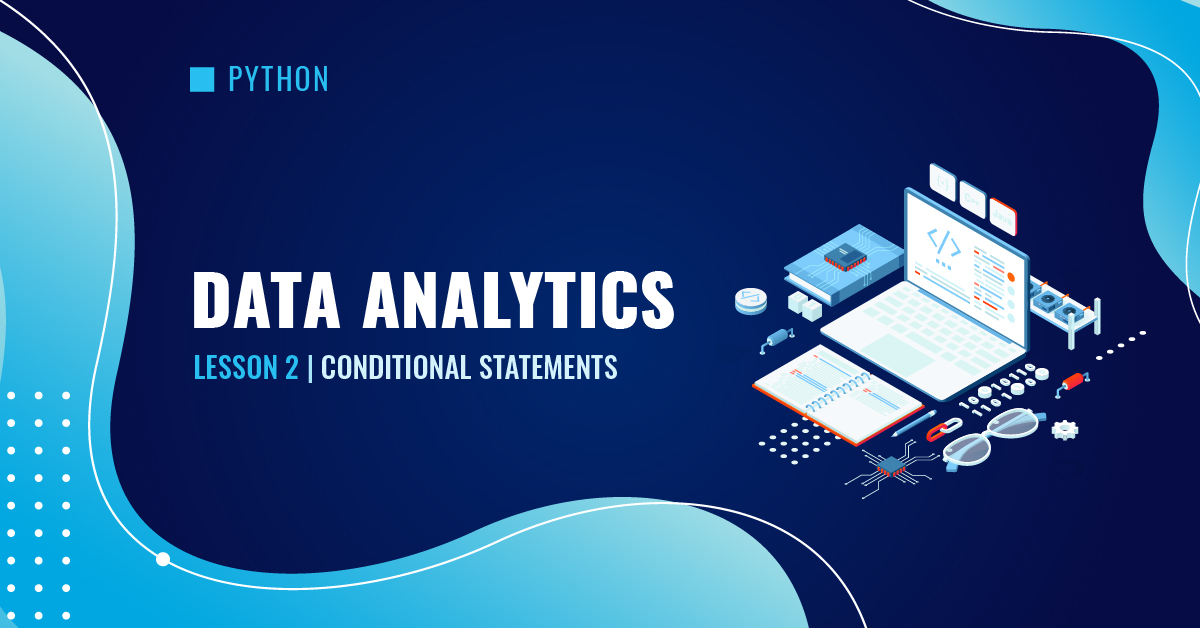Conditional statements are the basic building blocks of most programs coded in Python. They mimic human decision-making processes by asking the questions, “If this happens, what should be done?” and “If not, what should be done?”

## Logical Testing

Every condition involves a logical test (for example, whether a variable is equal to a certain value). Below is a list of the operators used for logical testing in Python.

Comparison operators compare two values and return a Boolean value.

Operator Description Example
==

Equal to

(Note: A single “=” assigns values to variables.)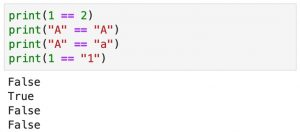!= Not equal to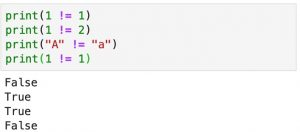> Greater than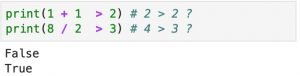< Less than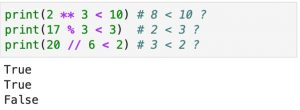>= Greater than or equal to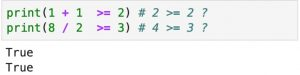<= Less than or equal to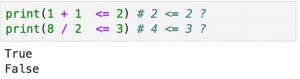is Is identical to (not equivalent to “equal to”)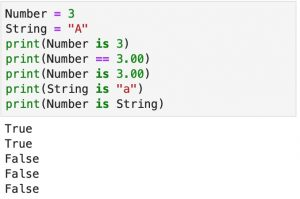is not Is not identical to (not equivalent to “not equal to”)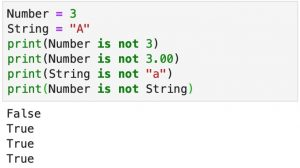Logical operators combine logical tests. It is recommended to put the logical tests in parentheses.

Operator Description Example

and

&

Returns True when all the conditions return True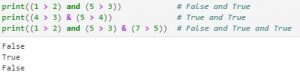or

|

Returns True when any condition returns True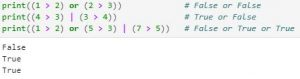not Negation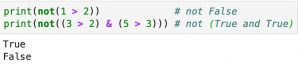Membership operators test if a value is in a set. For a dictionary, this tests whether a key (not a value) is in the dictionary.

Operator Description Example
in Returns True when the value is in the set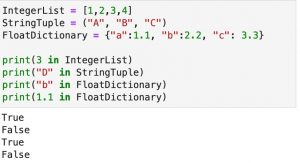not in Returns True when the value is not in the set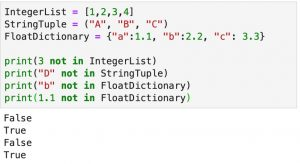## If Statements

### Single Condition

The simplest conditional statement consists only of an   if   statement followed by the operation specified in the   Tab   indented line. If the logical test is True, the operation will be performed; if not, nothing will happen.

if Logical Test:
Operation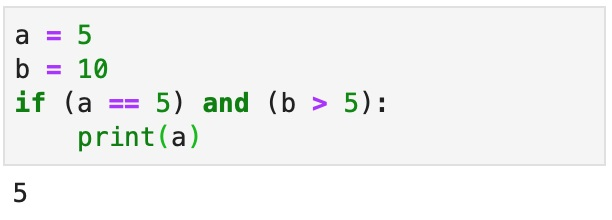### Leveled Condition

The level of indentation determines whether the indented code is executed.

Example 1: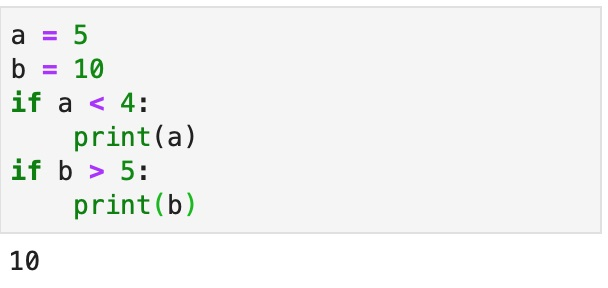The first   if   statement is false, so the operation to print variable “a” is not executed. The second   if   statement is a separate conditional statement. Because it is True, the operation to print variable “b” is executed.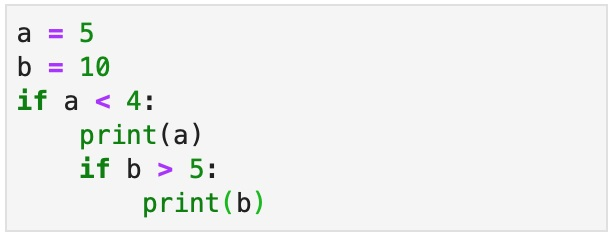The second   if   statement is subordinate to the first   if   statement. If the first   if   statement is not executed, the second   if   statement will not be evaluated.

The first   if   statement is false, so the operation to print variable “a” and the evaluation of the second   if   statement are not performed. No result is returned.

### Binary Selection

The   if-else   statement performs a binary selection of the operation to be performed. If the logical test in the   if   statement is True, the operation under   if   is executed; if not, the operation under   else   will be executed.

The following example compares two variables and prints the larger one. If variable “a” is greater than variable “b,” it prints variable “a.” If variable “b” is less than or equal to variable “a,” it prints variable “b.”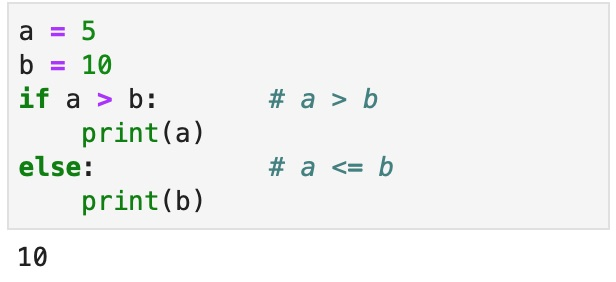While the example above is logically correct, showing only the variable without an explanation may sometimes be difficult to interpret. Adding a text explanation, such as “the larger value between 5 and 10 is 10,” can be used to help clarify the operation. A simple way to add explanations is to use   f-strings  .

f-strings   are a type of string in Python that is preceded by the letter   f  . When text is formatted as an   f-string  , the value stored in a variable can be displayed inside the string by enclosing the variable in curly brackets.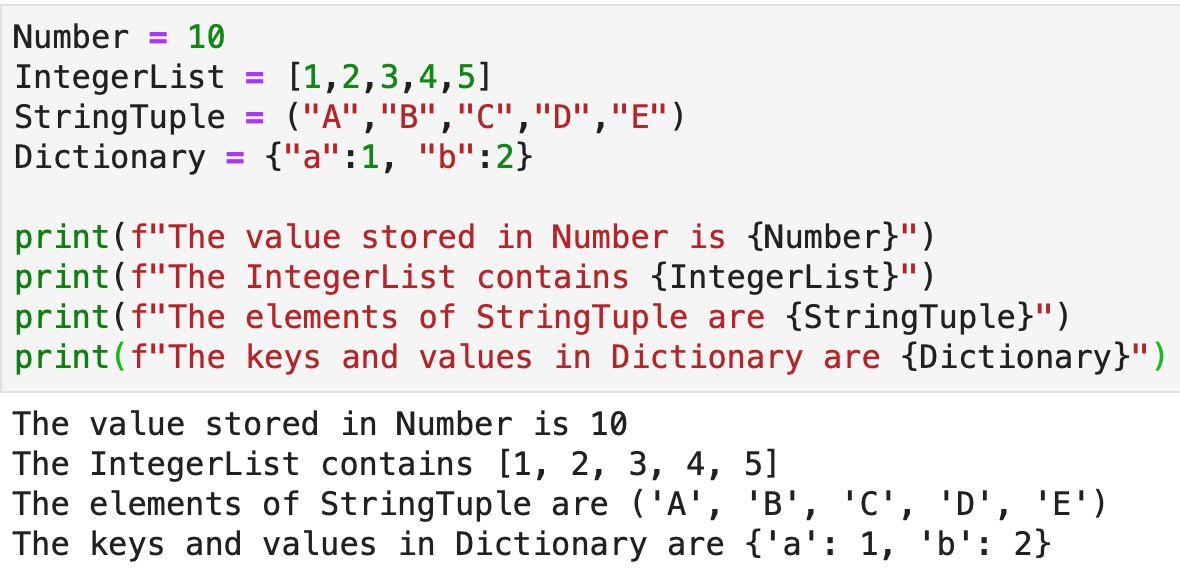Therefore, the earlier example to find the larger value between two variables can be rewritten as follows:### Multiple Conditions

If there are more than two conditions to be evaluated, the   if-else   combination is no longer sufficient. The   elif   (else if) statement can be included between the   if   statement and the   else   statement to add further conditions.

Example 1:

The following example compares the mark of a student against the ranges of the marks and returns the grade of the student.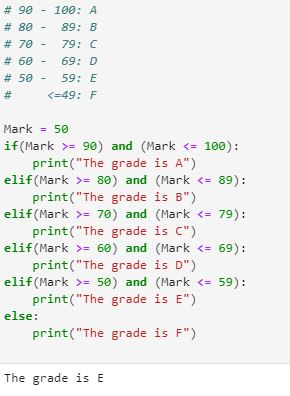Example 2:

The following example compares three variables and returns the largest value among them.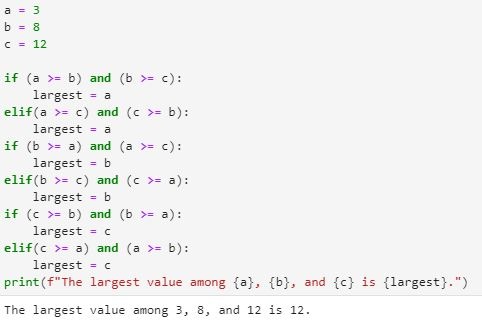Version A considers all six conditions and tests each of them using one if statement and four elif statements.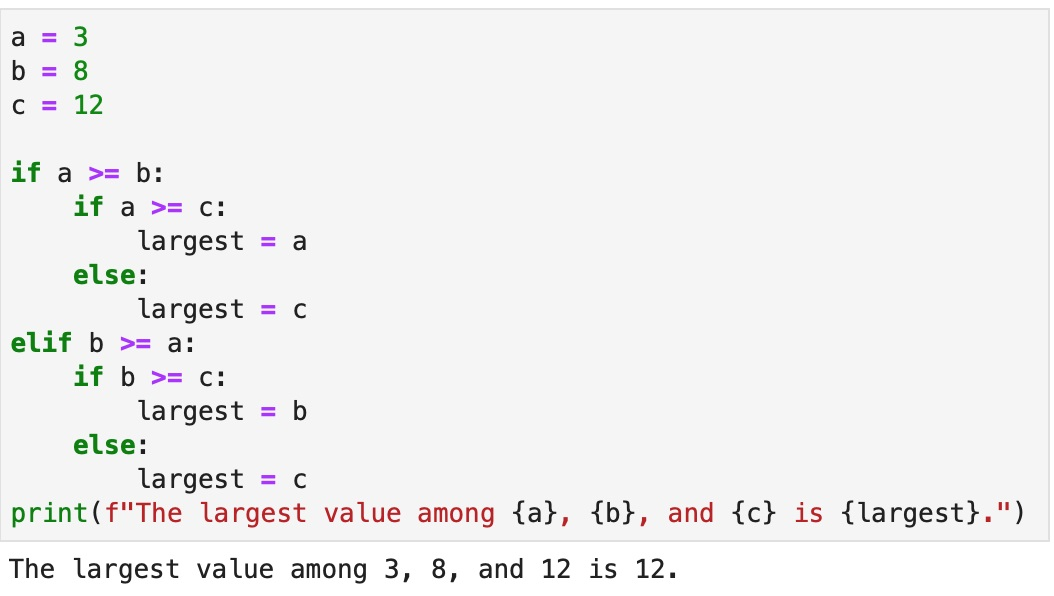Version B is a series of nested if and else statements. If a >= b and a >= c, a is the largest number; if a >= b and a < c, c is the largest number. Similarly, if b >= a and b >= c, b is the largest number; and if b >= a and b < c, c is the largest number.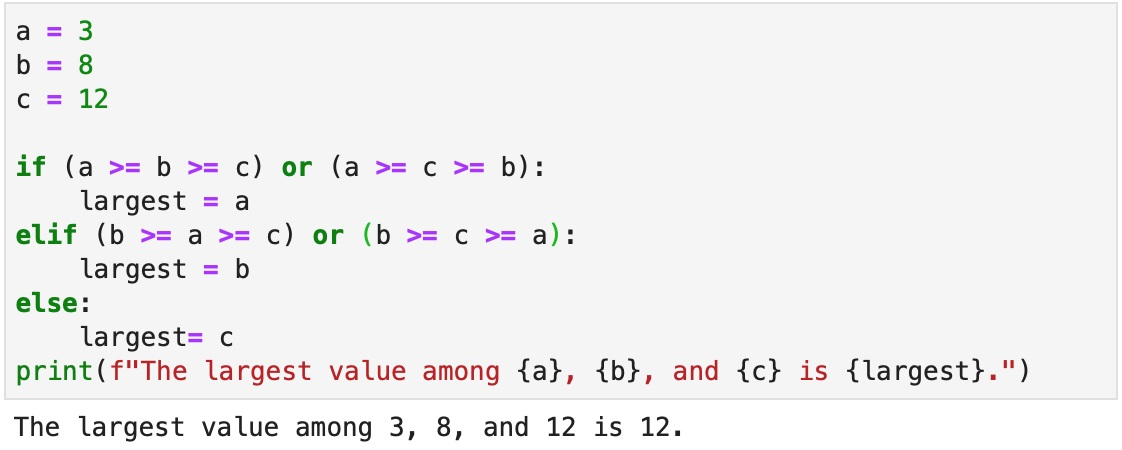Version C clusters the six conditions into three groups: (1) when variable “a” is the largest, (2) when variable “b” is the largest, and (3) when variable “c” is the largest. If the conditions in groups (1) and (2) are not True, the largest number must be variable “c.”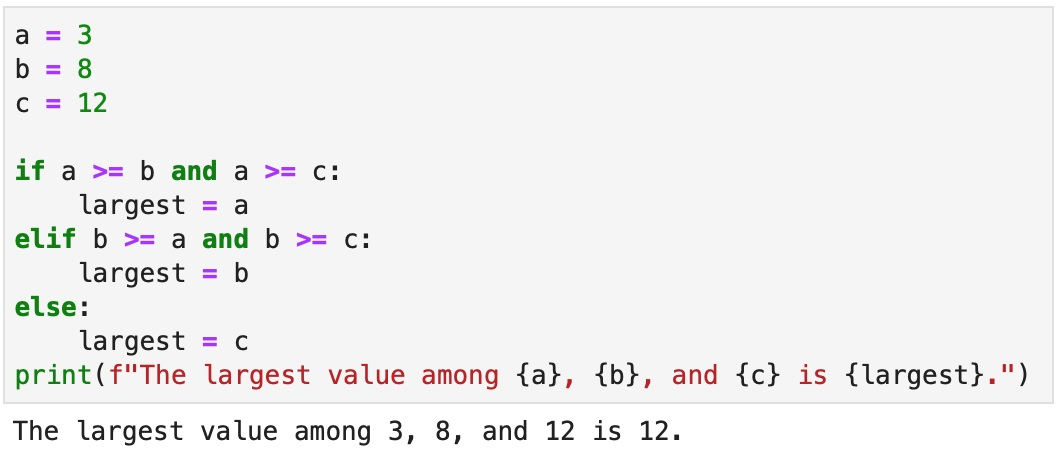Version D is the most intuitive. If   a >= b >= c   or   a >= c >= b  , variable “a” must be the largest among the three. However, this version is less effective when there are more variables. For example, when there are four variables, the conditions in which variable “a” is the largest are:
(1) a >= b >= c >= d
(2) a >= b >= d >= c
(3) a >= c >= b >= d
(4) a >= c >= d >= b
(5) a >= d >= b >= c
(6) a >= d >= c >= b

## Exercises:

1. For the three variables a = 4, b = 12, and c = 21, use conditional statements to find the median (middle number) among the three variables.

### Click for Solution

2. For the three variables a = 4, b = 12, and c = 21, use conditional statements to find the average of the largest and the smallest variables.

### Click for Solution

3. For the three variables a = 4, b = 12, and c = 21, use conditional statements to find the numbers that are both even and divisible by 3. Append the number to an empty list named “TheList.”

Hint: The remainder operator % returns the remainder when a number is divided by another number.

### Learning Python Data Analytics with Gleim

At Gleim, we know learning data analytics is vital for future certified accountants. That is why we are offering this series of data analytics blogs and continually updating all of our review materials for CPA, CMA, and CIA with the necessary information you need to pass your exam the first time!

We’ll continue our weekly blog series. Check back regularly for all exam news and study tips!

Python Lessons
Python Basics
Conditional Statements
Loops
Functions and Modules
Numerical Python (NumPy)
Pandas Basics
Pandas Data Capture and Cleansing
Merging and Grouping
Manipulating Text and Datetime Data
Visualization
Web Scraping
Errors and Exceptions